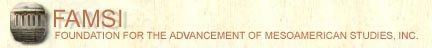John Montgomery
Dictionary of Maya Hieroglyphs

﻿

T

To hear the sounds and learn more, click on the text below the glyph.

Search the dictionary for words in English or Maya.

A | B' | CH | CH' | E | H | I | J | K | K' | L | M | N | O | P | S | T | T' | TZ | TZ' | U | W | X | Y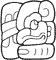TA OCH-le-{l} (ta ochlel)
(T102.765:188)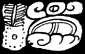TA yu-ta-{l} (ta yutal)
(T51.61:565)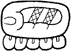ta-ji (taj)
(T565v:136)ta-ki (-tak)
(T565v.102)ta-ki-ja (takaj)
(T565:102.181)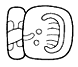ta-li (tal)
(T113.82)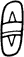ta/TA (ta)
(T103)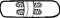ta/TA (ta)
(T113)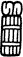ta/TA (ta)
(T122:150)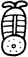ta/TA (ta)
(T51 and T53)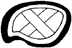ta/TA (ta)
(T552v)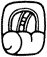ta/TA (ta)
(T565)ta/TA (ta)
(T565)ta/TA (ta)
(T565)ta/TA (ta)
(T645)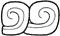ta/TA (ta)
(T776.776)TAJ (taj)
(T122:150)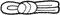TAJ (taj)
(T60)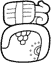TAJ TUN (taj tun)
(T122.150:528)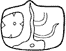TAK (-tak)
(T559)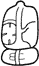TAK-ki (-tak)
(T559:102)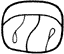TAL (tal)
(T676)TAN (tan)
(T606v)TAN (tan)
(T606)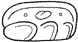TAN (tan)
(T606)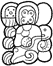TAN CH'EN OX WITZ-aj (tan ch'en ox witzaj)
(T606.571v:III.528hv.228)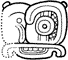TAN CH'EN-na (tan ch'en)
(T606.598v:23)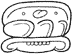TAN-na (tan)
(T606:23)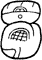te'-k'a (tek')
(T580:669)te'-k'a-ja (te' k'aj)
(T580:669b.181)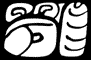TE'-le (te'el)
(T87:513.188)te/TE'/CHE' (te/te'/che')
(T350)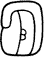te/TE'/CHE' (te/te'/che')
(T513)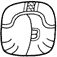te/TE'/CHE' (te/te'/che')
(T514)te/TE'/CHE' (te/te'/che')
(T514v)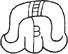te/TE'/CHE' (te/te'/che')
(T514v)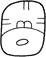te/TE'/CHE' (te/te'/che')
(T580)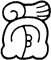te/TE'/CHE' (te/te'/che')
(T78:514v)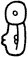te/TE'/CHE' (te/te'/che')
(T87)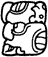te/TE'/CHE' (te/te'/che')
(T87hv)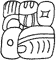TI AJAW le-{l} (ti ajawlel)
(T59.168:188)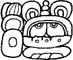TI ya-AK'-il (ti yakil?)
(T59.126:504v:24)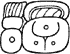ti-ka-la (tikal)
(T59.25:534)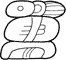ti-ki-li (tikil)
(T59:102:24)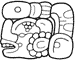ti-xu-[ku]-pi (ti xukpi)
(T59:756.177)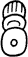ti/TI (ti)
(T59)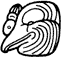ti/TI (ti)
(T747)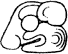TIL (til)
(T758v)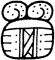to (to)
(T44:563b)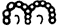to (to)
(T44)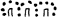to (to)
(T44)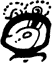to-jo-li (tojol)
(T44:607:24v)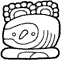to-jo-li (tojol)
(T44:695:24)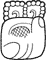to-k'a (tok')
(T44:669:314)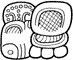to-k'a pa-ka-la (tok' pakal)
(T44:669.586b:25:178)to-ko (tok)
(T44:110)to-ko TAN AJAW (Tok Tan Ajaw)
(T110.168:44:606)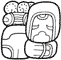to-ko TAN WINIK-ki (Tok Tan Winik)
(T44:110:606.521:102)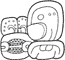to-ko-TAN-na (tok tan/Tok Tan)
(T44:110.606:23)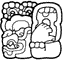to-XAT (toxat)
(T1030j)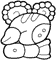to-[xa]-AT-ti (toxat)
(T44:691v:59)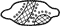TOK' (tok')
(T257)TOK' (tok')
(T257)TOK' (tok')
(T257)TOK' PAKAL (tok' pakal)
(T257:624)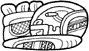TU K'U-li (tuk'ul)
(T89.1016:24)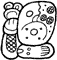TU WAY-[b'i]-li (tu wayb'il)
(T89.539:82)tu-ku-nu (tukun)
(T89.528:592v)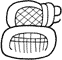tu-pa (tup)
(T89:586)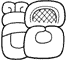tu-pa-ja (tupaj)
(T89.586:683)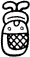tu/TU (tu)
(T89, T90, and T92)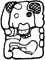TUN (tun)
(T1034)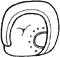TUN (tun)
(T528)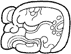TUN (tun)
(T528hv)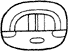TUN (tun)
(T548)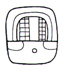TUN (tun)
(T548)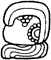TUN-ni (tun)
(T528:116)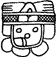[TAN] LAM (tan lam)
(T699v)[TAN] LAM-ja (tan lamaj)
(T699v.181)
Index | Next Page
Return to top of page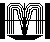Order John Montgomery’s Book